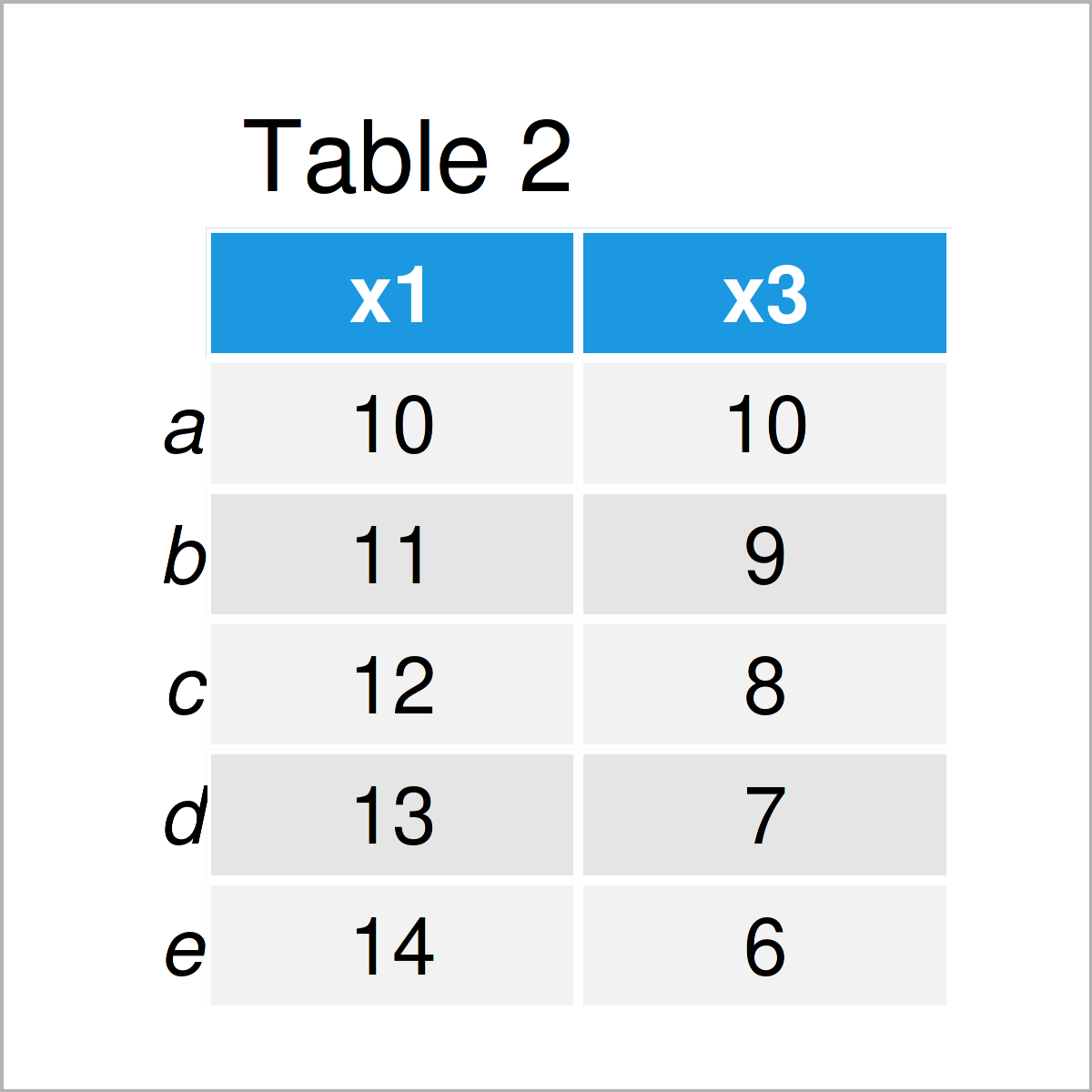# Set Index of pandas DataFrame in Python (Example)

In this Python programming article you’ll learn how to add a column of a pandas DataFrame as an index.

Let’s take a look at some Python codes in action.

## Example Data & Libraries

If we want to use the functions of the pandas library, we first have to load pandas to Python:

`import pandas as pd                         # Load pandas library`

The following data is used as a basis for this Python tutorial:

```data = pd.DataFrame({'x1':range(10, 15),    # Create pandas DataFrame
'x2':['a', 'b', 'c', 'd', 'e'],
'x3':range(10, 5, - 1)})
print(data)                                 # Print pandas DataFrame```Table 1 shows that our exemplifying data contains five rows and three columns. The index ranges from the value 0 to the number of rows in our data set.

## Example: Set Column as pandas DataFrame Index Using set_index() Function

In this example, I’ll illustrate how to use a column of a pandas DataFrame as an index in Python.

To achieve this, we have to apply the set_index function as shown below:

```data_new = data.set_index('x2')             # Apply set_index function
print(data_new)                             # Print updated DataFrame```The output of the previous Python programming syntax is shown in Table 2 – A new pandas DataFrame where the column x2 has been added as the index.

## Video & Further Resources

If you need further information on the Python code of this tutorial, I recommend having a look at the following video on my YouTube channel. I illustrate the Python code of the present tutorial in the video.

Please accept YouTube cookies to play this video. By accepting you will be accessing content from YouTube, a service provided by an external third party.If you accept this notice, your choice will be saved and the page will refresh.

Besides that, you may want to have a look at the related tutorials on my website:

You have learned in this tutorial how to use and add a column of a pandas DataFrame as an index in the Python programming language. Please let me know in the comments section, in case you have any further questions.

Subscribe to the Statistics Globe Newsletter